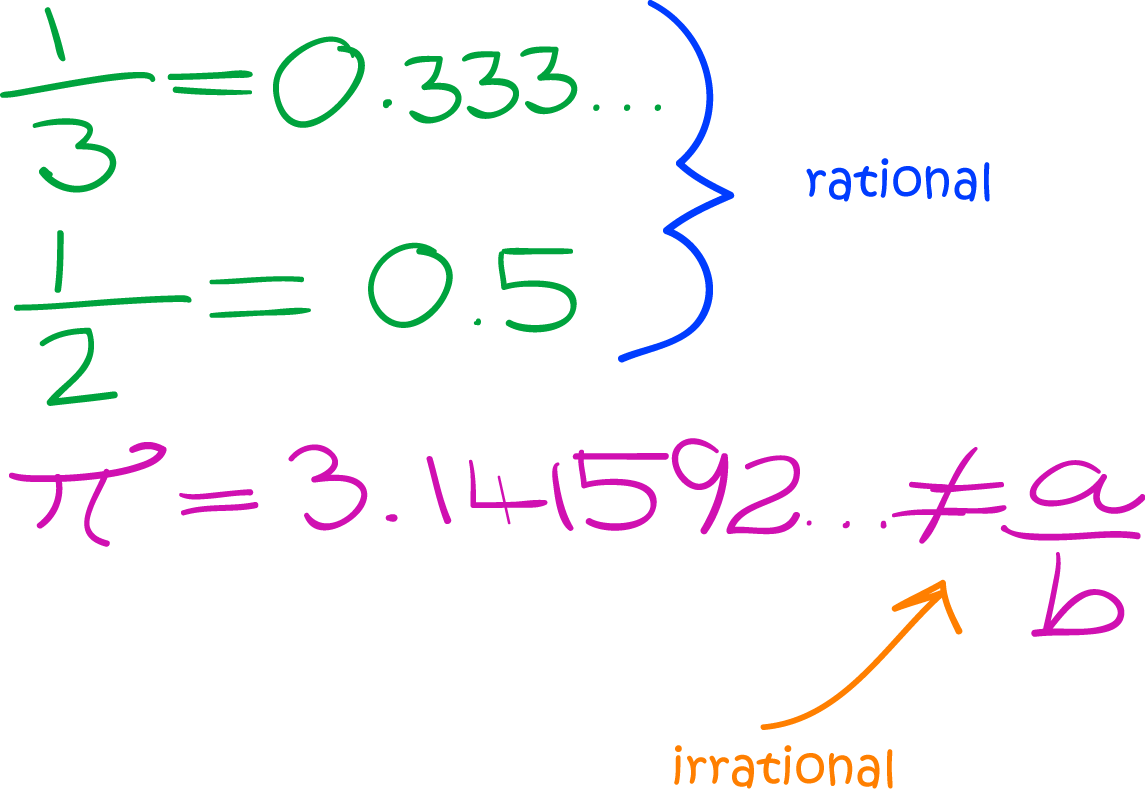# Definition of Irrational NumberAn irrational number is a number that cannot be written as a terminating or repeating decimal.

In other words, an irrational number is a number that can't be written as $\dfrac{a}{b}$ for some integers, $a$ and $b$.

For example, $\pi$ is an irrational number. Its digits are $3.141592\dots$. They go on forever, but don't form any pattern that repeats.

Some other examples of irrational numbers are $\sqrt{2}$ and Euler's number, $e = 2.71828\dots$.

### Description

The aim of this dictionary is to provide definitions to common mathematical terms. Students learn a new math skill every week at school, sometimes just before they start a new skill, if they want to look at what a specific term means, this is where this dictionary will become handy and a go-to guide for a student.

### Audience

Year 1 to Year 12 students

### Learning Objectives

Learn common math terms starting with letter I

Author: Subject Coach
You must be logged in as Student to ask a Question.